# Pinch (plasma physics)

Pinch (plasma physics)Lightning bolts illustrating electromagnetically pinched plasma filaments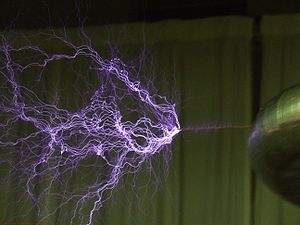Z-pinches constrain the plasma filaments in an electrical discharge from a Tesla coil. (Click to enlarge image for detail)

A pinch is the compression of an electrically conducting filament by magnetic forces. The conductor is usually a plasma, but could also be a solid or liquid metal. In a z-pinch, the current is axial (in the z direction in a cylindrical coordinate system) and the magnetic field azimuthal; in a theta-pinch, the current is azimuthal (in the theta direction in cylindrical coordinates) and the magnetic field is axial. The phenomenon may also be referred to as a "Bennett pinch" (after Willard Harrison Bennett), "electromagnetic pinch", "magnetic pinch", "pinch effect" or "plasma pinch".

Pinches occur naturally in electrical discharges such as lightning bolts, the aurora, current sheets, and solar flares. They are also produced in the laboratory, primarily for research into fusion power, but also by hobbyists (crushing aluminum cans).[citation needed]

## Pinch production and typesA section of the crushed lightning rod studied by Pollock and Barraclough. The rod is in the collection of the School of Physics, University of Sydney, Australia.

Pinches are created in the laboratory in equipment related to nuclear fusion, such as the Z-pinch machine, and high-energy physics, such as the dense plasma focus. Pinches may also become unstable, and generate radiation across the electromagnetic spectrum, including radio waves, x-rays and gamma rays, and also neutrons and synchrotron radiation. Types of pinches, that may differ in geometry and operating forces, include the cylindrical pinch, inverse pinch, orthogonal pinch effect, reversed field pinch, sheet pinch, screw pinch (also called stabilized z-pinch, or θ-z pinch), theta pinch (or thetatron), toroidal pinch, ware pinch and Z-pinch.

Pinches are used to generate X-rays, and the intense magnetic fields generated are used in electromagnetic forming of metals (they have been demonstrated in crushing aluminium soft drinks cans). They have applications to particle beams including particle beam weapons, and astrophysics.

## HistoryThe Institute of Electrical and Electronics Engineers emblem shows the basic features of an azimuthal magnetic pinch.

The first creation of a z-pinch in the laboratory may have occurred in 1790 in Holland when Martinus van Marum created an explosion by discharging 100 Leyden jars into a wire. The phenomenon was not understood until 1905, when Pollock and Barraclough investigated a compressed and distorted length of copper tube from a lightning rod after it had been struck by lightning. Their analysis showed that the forces due to the interaction of the large current flow with its own magnetic field could have caused the compression and distortion. A similar, and apparently independent, theoretical analysis of the pinch effect in liquid metals was published by Northrupp in 1907. The next major development was the publication in 1934 of an analysis of the radial pressure balance in a static z-pinch by Bennett (See the following section for details.)

Thereafter, the experimental and theoretical progress on pinches was driven by fusion power research. In their article on the "Wire-array z-pinch: a powerful x-ray source for ICF", M G Haines et al., wrote on the "Early history of z-pinches":

In 1946 Thompson and Blackman  submitted a patent for a fusion reactor based on a toroidal z-pinch  with an additional vertical magnetic field. But in 1954 Kruskal and Schwarzschild  published their theory of MHD instabilities in a z-pinch. In 1956 Kurchatov gave his famous Harwell lecture showing nonthermal neutrons and the presence of m = 0 and m = 1 instabilities in a deuterium pinch . In 1957 Pease  and Braginskii  independently predicted radiative collapse in a z-pinch under pressure balance when in hydrogen the current exceeds 1.4 MA. (The viscous rather than resistive dissipation of magnetic energy discussed above and in  would however prevent radiative collapse). Lastly, at Imperial College in 1960, led by R Latham, the Plateau-Rayleigh instability was shown, and its growth rate measured in a dynamic z-pinch ."

## Configurations

### One-dimensional configurations

There are three analytic one dimensional configurations generally studied in plasma physics. These are the θ-pinch, the Z-pinch, and the Screw Pinch. All of the classic one dimensional pinches are cylindrically shaped. Symmetry is assumed in the axial (z) direction and in the azimuthal (θ) direction. It is traditional to name a one-dimensional pinch after the direction in which the current travels.

The θ-pinch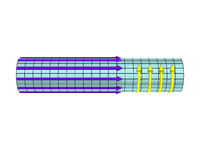A sketch of the θ-Pinch Equilibrium. The z directed magnetic field (shown in purple) corresponds to a θ directed plasma current (shown in yellow).

The θ-pinch has a magnetic field traveling in the z direction. Using Ampère's law (discarding the displacement term)$\nabla \times \vec{B} = \mu_0 \vec{J}$$\vec{B} = B_{z}(r)\hat{z}$$\mu_{0} \vec{J} = \frac{d}{d \theta}B_z \hat{r} - \frac{d}{dr}B_z \hat{\theta}$

Since B is only a function of r we can simplify this to$\mu_0 \vec{J} = -\frac{d}{dr}B_z \hat{\theta}$

So J points in the θ direction. θ-pinches tend to be resistant to plasma instabilities. This is due in part to the frozen in flux theorem, which is beyond the scope of this article.

The Z-Pinch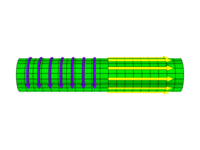A sketch of the z-Pinch Equilibrium. A -θ directed magnetic field (shown in purple) corresponds to a z directed plasma current (shown in yellow).

The Z-Pinch has a magnetic field in the θ direction. Again, by electrostatic Ampere's Law$\nabla \times \vec{B} = \mu_0 \vec{J}$$\vec{B} = B_{\theta}(r)\hat{\theta}$$\mu_{0} \vec{J} = \frac{1}{r}\frac{d}{dr}(r B_{\theta}) \hat{z} - \frac{d}{dz}B_{\theta} \hat{r}$$\mu_{0} \vec{J} = \frac{1}{r}\frac{d}{dr}(r B_{\theta}) \hat{z}$

So J points in the z direction. Since particles in a plasma basically follow magnetic field lines, Z-pinches lead them around in circles. Therefore, they tend to have excellent confinement properties.

The screw pinch The screw pinch is an effort to combine the stability aspects of the θ-pinch and the confinement aspects of the Z-pinch. Referring once again to Ampere's Law$\nabla \times \vec{B} = \mu_0 \vec{J}$

But this time, the B field has a θ component and a z component$\vec{B} = B_{\theta}(r)\hat{\theta} + B_z (r) \hat{z}$$\mu_0 \vec{J} = \frac{1}{r}\frac{d}{dr}(r B_{\theta}) \hat{z} - \frac{d}{dr}B_{z} \hat{\theta}$

So this time J has a component in the z direction and a component in the θ direction.

### Two-dimensional equilibria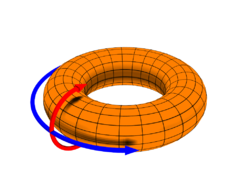A toroidal coordinate system in common use in plasma physics. The red arrow indicates the poloidal direction (θ) and the blue arrow indicates the toroidal direction (φ)

A common problem with one-dimensional equilibria based machines is end losses. As mentioned above, most of the motion of particles in a plasma is directed along the magnetic field. With the θ-pinch and the screw-pinch, this leads particles to the end of the machine very quickly (as the particles are typically moving quite fast). Additionally, the Z-pinch has major stability problems. Though particles can be reflected to some extent with magnetic mirrors, even these allow many particles to pass. The most common method of mitigating this effect is to bend the cylinder around into a torus. Unfortunately this breaks θ symmetry, as paths on the inner portion (inboard side) of the torus are shorter than similar paths on the outer portion (outboard side). Thus, a new theory is needed. This gives rise to the famous Grad–Shafranov equation.

The one dimensional equilibria provide the inspiration for some of the toroidal configurations. An example of this is the ZETA device at Culham England (which also operated as a Reversed Field Pinch). The most well recognized of these devices is the toroidal version of the screw pinch, the Tokamak.

Numerical solutions to the Grad–Shafranov equation have also yielded some equilibria, most notably that of the reversed field pinch.

### Three-dimensional equilibria

There does not exist a coherent analytical theory for three-dimensional equilibria. The general approach to finding three dimensional equilibria is to solve the vacuum ideal MHD equations. Numerical solutions have yielded designs for stellarators. Some machines take advantage of simplification techniques such as helical symmetry (for example University of Wisconsin's Helically Symmetric eXperiment).

## Formal treatment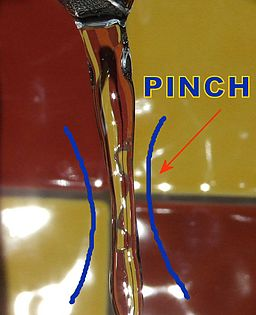A stream of water pinching into droplets has been suggested as an analogy to the electromagnetic pinch. The gravity accelerates free-falling water which causes the water column to constrict. Then surface tension breaks the narrowing water column into droplets (not shown here) (see Plateau-Rayleigh instability), which is analogous to the magnetic field which has been suggested as the cause of pinching in bead lightning. The morphology (shape) is similar to the so-called sausage instability in plasma.

### The Bennett relation

Consider a cylindrical column of fully ionized quasineutral plasma, with an axial electric field, producing an axial current density, j, and associated azimuthal magnetic field, B. As the current flows through its own magnetic field, a pinch is generated with an inward radial force density of j x B. In a steady state with forces balancing:

p = ∇(pe + pi) = j × Β

where ∇p is the magnetic pressure gradient, pe and pi is the electron and ion pressures. Then using Maxwell's equation ∇ × B = μ0 j and the ideal gas law p = N k T, we derive:$2 N k(T_e + T_i) = \frac{{\mu_0}} {4 \pi} I^2$ (the Bennett relation)

where N is the number of electrons per unit length along the axis, Te and Ti are the electron and ion temperatures, I is the total beam current, and k is the Boltzmann constant.

### The generalized Bennett relation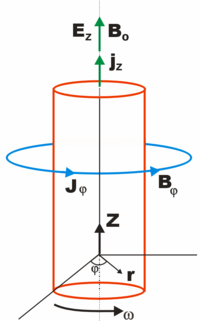The generalized Bennett relation considers a current-carrying magnetic-field-aligned cylindrical plasma pinch undergoing rotation at angular frequency ω

The Generalized Bennett Relation considers a current-carrying magnetic-field-aligned cylindrical plasma pinch undergoing rotation at angular frequency ω. Along the axis of the plasma cylinder flows a current density jz, resulting in a toroidal magnetίc field Βφ. Originally derived by Witalis, the Generalized Bennett Relation results in:\begin{align} \frac{1}{4} \frac{\partial^2 J_0}{\partial t^2} & = W_{\perp \text{kin}} + \Delta W_{E_z} + \Delta W_{B_z} + \Delta W_k - \frac{{\mu_0}} {8 \pi} I^2 (a) \\[8pt] & {} - \frac{1}{2}G\overline{m}^2 N^2 (a) + \frac{1}{2}\pi a^2 \epsilon_0 \left(E_r^2 (a) - E_\phi^2 (a) \right) \end{align}
• where a current-carrying, magnetic-field-aligned cylindrical plasma has a radius a,
• J0 is the total moment of inertia with respect to the z axis,
• W⊥kin is the kinetic energy per unit length due to beam motion transverse to the beam axis
• WBz is the self-consistent Bz energy per unit length
• WEz is the self-consistent Ez energy per unit length
• Wk is thermokinetic energy per unit length
• I(a) is the axial current inside the radius a (r in diagram)
• N(a) is the total number of particles per unit length
• Er is the radial electric field
• Eφ is the rotational electric field

The positive terms in the equation are expansional forces while the negative terms represent beam compressional forces.

### The Carlqvist relation

The Carlqvist Relation, published by Per Carlqvist in 1988, is a specialization of the Generalized Bennett Relation (above), for the case that the kinetic pressure is much smaller at the border of the pinch than in the inner parts. It takes the form$\frac{{\mu_0}} {8 \pi} I^2 (a) +\frac{1}{2}G\overline{m}^2 N^2 (a) = \Delta W_{B_z} + \Delta W_k$

and is applicable to many space plasmas.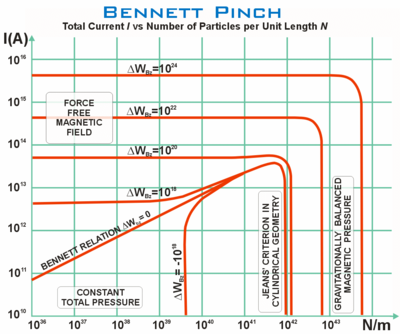The Bennett pinch showing the total current (I) versus the number of particles per unit length (N). The chart illustrates four physically distinct regions. The plasma temperature is 20 K, the mean particle mass 3×10−27 kg, and ΔWBz is the excess magnetic energy per unit length due to the axial magnetic field Bz. The plasma is assumed to be non-rotational, and the kinetic pressure at the edges is much smaller than inside.

The Carlqvist Relation can be illustrated (see right), showing the total current (I) versus the number of particles per unit length (N) in a Bennett pinch. The chart illustrates four physically distinct regions. The plasma temperature is quite cold (Ti = Te = Tn = 20 K), containing mainly hydrogen with a mean particle mass 3×10−27 kg. The thermokinetic energy Wk >> πa2 pk(a). The curves, ΔWBz show different amounts of excess magnetic energy per unit length due to the axial magnetic field Bz. The plasma is assumed to be non-rotational, and the kinetic pressure at the edges is much smaller than inside.

Chart regions: (a) In the top-left region, the pinching force dominates. (b) Towards the bottom, outward kinetic pressures balance inwards magnetic pressure, and the total pressure is constant. (c) To the right of the vertical line ΔWBz = 0, the magnetic pressures balances the gravitational pressure, and the pinching force is negligible. (d) To the left of the sloping curve ΔWBz = 0, the gravitational force is negligible. Note that the chart shows a special case of the Carlqvist relation, and if it is replaced by the more general Bennett relation, then the designated regions of the chart are not valid.

Carlqvist further notes that by using the relations above, and a derivative, it is possible to describe the Bennett pinch, the Jeans criterion (for gravitational instability, in one and two dimensions), force-free magnetic fields, gravitationally balanced magnetic pressures, and continuous transitions between these states.

## Crushing cans with the pinch effectPinched aluminium can, produced from a pulsed magnetic field created by rapidly discharging 2 kilojoules from a high voltage capacitor bank into a 3-turn coil of heavy gauge wire.

Many high-voltage electronics enthusiasts make their own devices using pulsed power techniques to produce a theta pinch capable of crushing an aluminium soft drink can by pressure of strong magnetic field.

An electromagnetic aluminium can crusher consists of four main components (1) A high voltage DC power supply which provides a source of electrical energy (2) A large energy discharge capacitor to accumulate the electrical energy (3) A high voltage switch or spark gap and (4) A robust coil (capable of surviving high magnetic pressure) through which the stored electrical energy can be quickly discharged in order to generate a correspondingly strong pinching magnetic field (see diagram below).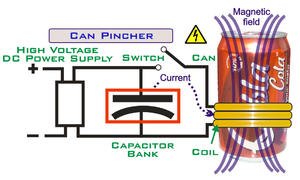Electromagetic pinch "can crusher": schematic diagram

In practice, such a device is somewhat more sophisticated than the schematic diagram suggests, including electrical components that control the current in order to maximize the resulting pinch, and to ensure that the device works safely. For more details, see the notes.

Sam Barros's can crusher cost about \$500, and uses a large SCR and a 900 Volt capacitor bank storing about 3000 Joules of energy. For a very short time, it generates a magnetic field B~5T (250,000 times the strength of the Earth's magnetic field) which has magnetic pressure P ~ 100 atm. Rate of energy conversion (from electric into magnetic and back) in this device is about 22 megawatts.

## Depictions

A fictionalized pinch-generating device was used in Ocean's Eleven, where it was used to disrupt Las Vegas's power grid just long enough for the characters to begin their heist.

Wikimedia Foundation. 2010.

### Look at other dictionaries:

• Plasma (physics) — For other uses, see Plasma. Plasma lamp, illustrating some of the more complex phenomena of a plasma, including filamentation. The colors are a result of relaxation of electrons in excited states to lower energy states after they have recombined… …   Wikipedia

• Pinch — may refer to:* Pinch (cooking), a very small amount of an ingredient, typically salt or a spice * Pinch, West VirginiaMathematics and Science* Pinch (plasma physics), the compression of a plasma filament by magnetic forces, or a device which uses …   Wikipedia

• Plasma-Physik — Plasma in einer Plasmalampe. Datei:Gerds plasma.jpg magnetisch verformtes Plasma Sonne …   Deutsch Wikipedia

• Plasma (Physik) — Plasma in einer Plasmalampe Magnetisch verformtes Plasma …   Deutsch Wikipedia

• plasma — plasmatic /plaz mat ik/, plasmic, adj. /plaz meuh/, n. 1. Anat., Physiol. the liquid part of blood or lymph, as distinguished from the suspended elements. 2. Cell Biol. cytoplasm. 3. whey. 4. a green, faintly translucent chalcedony. 5. Physics. a …   Universalium

• Plasma stability — An important field of plasma physics is the stability of the plasma. It usually only makes sense to analyze the stability of a plasma once it has been established that the plasma is in equilibrium. Equilibrium asks whether there are net forces… …   Wikipedia

• pinch effect — Physics. the tendency of an electric conductor or stream of charged particles to constrict, caused by the action of a magnetic field that is produced by a flow of electricity. [1905 10] * * * ▪ physics       self constriction of a cylinder of an… …   Universalium

• pinch effect — n. Physics the constriction, or compression, of plasma by the action of the magnetic field of a strong electric current flowing through the plasma …   English World dictionary

• Dense plasma focus — Plasma gun redirects here. For the science fiction weapon, see plasma weapon (fiction). A dense plasma focus (DPF) is a machine that produces, by electromagnetic acceleration and compression, a short lived plasma that is so hot and dense that it… …   Wikipedia

• Reversed field pinch — A reversed field pinch (RFP) is a device used to produce and contain near thermonuclear plasmas. It is a toroidal pinch which uses a unique magnetic field configuration as a scheme to magnetically confine a plasma, primarily to study magnetic… …   Wikipedia